### Home > CALC > Chapter 6 > Lesson 6.2.1 > Problem6-59

6-59.
1. For each of the following equations, find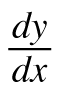. Homework Help ✎

1. y = x3 sin(cos x)

2. 3xyx2 = 2

3.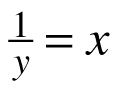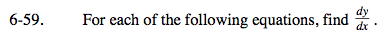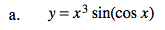Use both the Chain and Product Rule.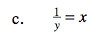Solve for y first.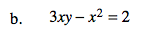Before you differentiate, you could solve for y.
Or, you could use implicit differentiation:

$\frac{d}{dx}(3xy-x^{2})=\frac{d}{dx}(2)$

$3y\left (\frac{dx}{dx} \right )+3x\left ( \frac{dy}{dx} \right )-2x\left ( \frac{dx}{dx} \right )=0$

$\text{Note: }\left ( \frac{dx}{dx} \right )=1$

$\text{Solve for }\left ( \frac{dy}{dx} \right ).$